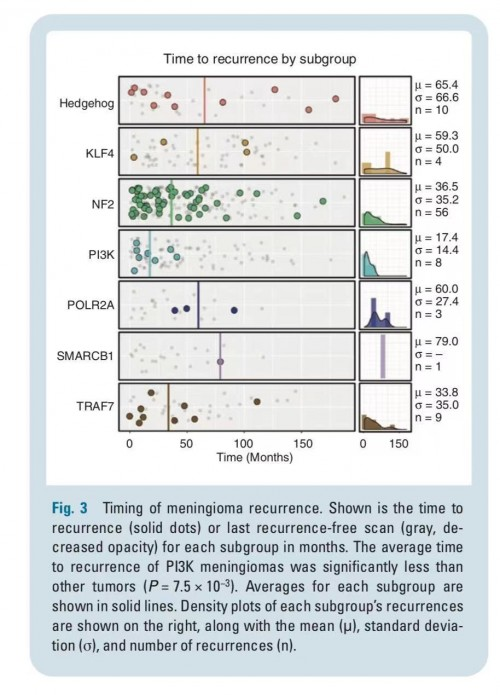复刻一篇文章里面的图片

一息尚存，此志不容少懈。

Posted by Chevy on May 24, 2021

原文图片附上代码

xl_data <- "mutation type.xlsx"
tab_names <- excel_sheets(path = xl_data)
list_all <- lapply(tab_names, function(x) read_excel(path = xl_data, sheet = x) %>% mutate(Tumor_Sample_Barcode = x))
df <- rbind.fill(list_all)

os <- read_delim(file = "overall_survival.txt", delim = "\t")
os\$jitter <- rnorm(nrow(os), mean = 1, sd = 0.2)

plot_dot <- function(name, n) {

barcode = df %>% filter(gene == name) %>% select(Tumor_Sample_Barcode) %>% unique()

p1 <- ggplot(os[os\$Pathological_number %in% barcode\$Tumor_Sample_Barcode,], aes(x = Progression_time,  y = jitter)) +
geom_point(shape = 21,size = 4, color = "black", fill = RColorBrewer::brewer.pal(n = 9, name = "Paired")[n], alpha = 0.7) +
geom_point(data = os[!os\$Pathological_number %in% barcode\$Tumor_Sample_Barcode,],
aes(x = Progression_time, y = jitter), shape = 21, fill = "grey", size = 2) +
geom_vline(xintercept = mean(os[os\$Pathological_number %in% barcode\$Tumor_Sample_Barcode,]\$Progression_time),
size = 2, color = RColorBrewer::brewer.pal(n = 9, name = "Paired")[n]) +
scale_x_continuous(expand = c(0, 0), limits = c(0, 140)) +
scale_y_continuous(expand = c(0, 0), limits = c(0, 2)) +
theme_bw(base_rect_size = 2) +
theme(axis.title = element_blank(),
axis.ticks = element_blank(),
axis.text = element_blank())

p2 <- ggplot(os[os\$Pathological_number %in% barcode\$Tumor_Sample_Barcode,], aes(x = Progression_time)) +
geom_histogram(aes(y = ..density..), binwidth = 10,
fill = RColorBrewer::brewer.pal(n = 9, name = "Paired")[n]) +
geom_density(fill = RColorBrewer::brewer.pal(n = 9, name = "Paired")[n],
alpha = 0.5, size = 1, outline.type = "full") +
scale_x_continuous(expand = c(0, 0)) +
theme_bw(base_rect_size = 2) +
theme(axis.title = element_blank(),
axis.ticks = element_blank(),
axis.text = element_blank())

plot_grid(p1, p2, rel_widths = c(10,2), labels = name)
}